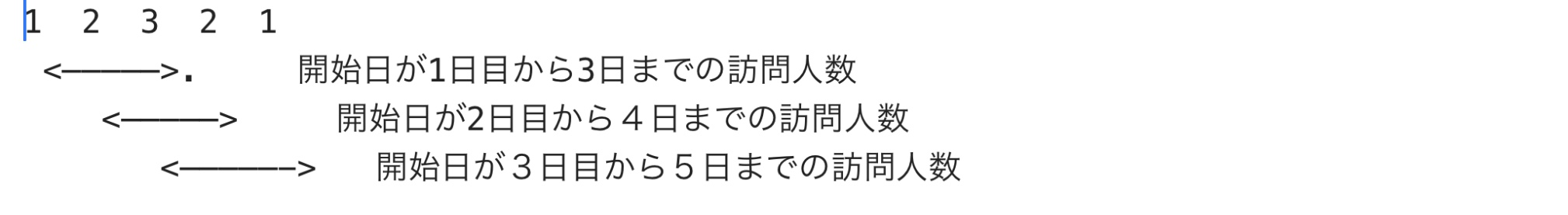# 0. Problem

The target total number of days is DD, and you will visit during a certain N days of the total number of days. The number of candidate days for the start date of the section where the average number of people is maximum, and among those candidate days Give the earliest day.Here is a summary of what I thought about in solving this problem. I was able to clear it by the following procedure.

# 1. Try to draw a diagram first

The following two lines are given.

``````Total number of days on the first line(DD)Number of days in the section you want to find(N)\\
Number of visitors for each day on the second line(A_i(i=1,,,DD))
``````

`5 3` `1 2 3 2 1`Thinking as above, On the second day of the start date, the number of daily visitors will be eight, which is the maximum average section. Also, since the number of visitors is maximized only when the start date is 2 days, the number of candidate days is It becomes 1. It turns out that the answer is "1 2".

# 2. Way of thinking (pattern division)

Here, consider that `the average is maximized = the sum of the number of daily visitors in a certain section is maximized`.

## `(1) When DD and N are the same`

⇒ There is no need to calculate the number of visitors to DD Since there is only one candidate date and section pattern, [1 1] is the answer.

## `(2) When DD> N and N> 1`

``````X_1=A_1+...+A_{N}\\
max1=X_1(max1:Maximum number of visitors in N days)\\
C_{day}=1(C_{day}:Number of candidate days)\\
S_{day}=d(S_{day}:Start date of maximum number of visitors in N days)
``````
``````X_d:Let d be the number of visitors for N days when the start date is
From the previous chapter, we can see the following.
With this recurrence formula, the number of calculations can be reduced.
``````
``````X_d=X_{d-1}-A_{d-1}+A_{d-1+N}(d=2,,,DD-N+1)-★\\

``````

### `I) Number of visitors for N days with a certain start date d> Processing of maximum number of visitors`

``````C_{day}=1\\
max1=X_d\\
S_{day}=d

``````

### `Ii) Number of visitors for N days with a certain start date d = Processing of maximum number of visitors`

``````C_{day}=C_{day}+1\\
max1=X_d

``````

## `(3) When DD> N and N = 1`

``````X_d=A_d(d=1,,,DD)
``````

Consider the processing of the conditions 1) and 2) in the case of (2) above.

# 3. Code example

``````
in1=input()
in2=input()
arr1=in1.split()
arr2=in2.split()

dn=0

num1=int(arr1)
#print(num1)

if num1>1:
i=0
for i in range(num1):
dn=dn+int(arr2[i])

max_t=dn
max_i=0
k_cnt=1

for i in range(1,int(arr1)-num1+1):
dn=dn+int(arr2[i+num1-1])-int(arr2[i-1])

if max_t<dn:
k_cnt=1
max_i=i
max_t=dn

else:
if max_t == dn:
k_cnt=k_cnt+1
max_t=dn

max_i=max_i+1
print(str(k_cnt)+' '+str(max_i))

elif num1>1 and num1 == int(arr1):
print('1 1')
else:
max_i=0
max_t=0
k_cnt=1
for i in range(int(arr1)):
dn=int(arr2[i])
if max_t<dn:
max_t=dn
k_cnt=1
max_i=i
else:
if max_t == dn:
max_t=dn
k_cnt=k_cnt+1

max_i=max_i+1
print(str(k_cnt)+' '+str(max_i))

``````